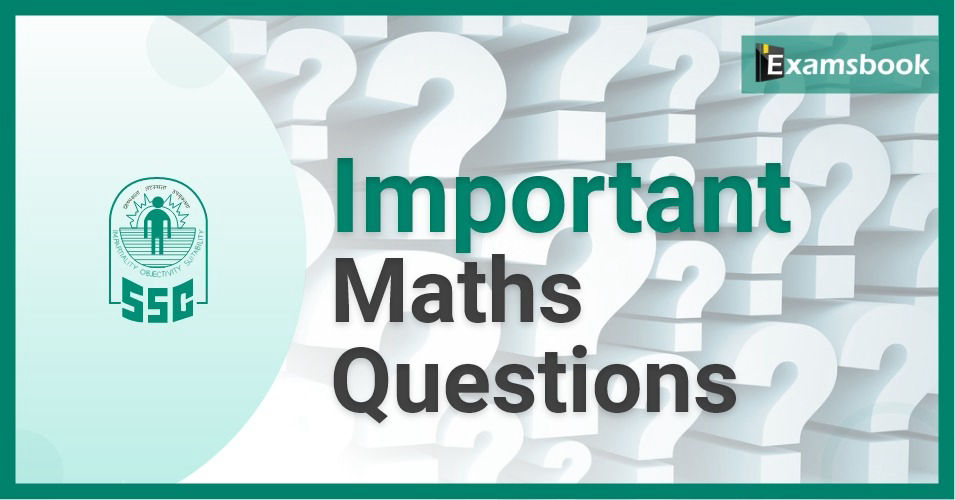• Save

# Important Maths Questions for SSC CGL ExamVery important Maths Questions for SSC CGL Exams. You can easily score extra with the help of Maths Questions for SSC CGL Exams.

Practice with these important Maths Questions for better results in exams in maths section. You should also check GK Questions for 2018 blog for complete GK Questions.

Here are related blogs Time and Distance and Important Questions of Profit and Loss for SSC CGL Exam.

## Important Maths questions for SSC CGL Exam

1.   The product of 2 numbers is 1575 and their quotient is 9/7. Then the sum of the numbers is –

a.   74

b.   78

c.   80

d.   90

Ans : Let the numbers be x and y .

We know   xy = 1575

And  x/y = 9/7

We know   xy/x/y = 1575/9/7

We know    y2  = 1225

We know  y = 35 and x = 45

We know   The sum of the numbers = 45+35

= 80

2.   The value of (81)3.6 * (9)2.7/ (81)4.2 * (3) is __

a.   3

b.   6

c.   9

d.   8.2

Ans : (81)3.6 * (9)2.7/(81)4.2 * (3) = (3)14.4 * (3)5.4/(3)16.8 * (3)

= 314.4+5.4-16.8-1

= 32

= 9

3.  √6+√6+√6+… is equal to –

a. 2
b. 5
c. 4
d. 3

Ans : Let √6+√6+√6+…. be x.
We know x =√6+x
We know x2 = 6+x
We know x2 - x – 6 = 0
We know (x-3) (x+2) = 0
We know x = 3

4.   The sum of the squares of two natural consecutive odd numbers is 394. The sum of the numbers is –

a.   24

b.   32

c.   40

d.   28

Ans : Let the consecutive odd numbers be x and (x+2)

We know that x2 + (x + 2)2 = 394

We know   x2 + x2 + 4x + 4 = 394

We know   2 x2 +4x – 390 = 0

We know   x2 + 2x – 195 = 0

We know  (x +15) (x-13) = 0

We know   x = 13

We know    Required sum = 13 +15 =28

5.   When (6767 +67) is divided by 68, the remainder is-

a.   1

b.   63

c.   66

d.   67

Ans:  (6767+ 67) = 67(6766 + 166)

As 66 is an even number

We know   6766 is an even number

We know   (6766 + 1) is perfectly divisible by (67 + 1)

i.e. 68

We know   The remainder = 66

6.   In a division sum, the divisor is 4 times the quotient and twice the remainder. If a and b are respectively the divisor and the dividend then-

a.   4b-a2 /a  =3

b.   4b-2a /a2 =2

c.   (a+1)2 = 4b

d.   A(a+2)/b = 4

Ans : As  divisor is a, and dividend is b.

We know    Quotient = a/4

And Remainder = a/2

We know    b = a * a/4 + a/2

We know    4b = a2 + 2a

We know    a(a+2)/b = 4

7.   If 738 A6A is divisible by 11, then the value of A is-

a.   6

b.   3

c.   9

d.   1

Ans :  As 738A6 A is divisible by 11.

We know    A + A + 3 = 6 + 8 + 7

We know    A = 9

8.   The east positive integer that should be subtracted from 3011 * 3012 so that the difference is perfect square is-

a.   3009

b.   3010

c.   3011

d.   3012

Ans :  We know that 3011 * 3012 = 3011 (3011 + 1)

= (3011)2 + 3011

We know    Required least number = 3011

9.   P, Q, R are employed to do a work for Rs. 5750. P and Q together finished 19/23 of work and Q and R together finished 8/23 of work. Wage of Q, in rupees, is-

a.   2850

b.   3750

c.   2750

d.   1000

Ans :  Work done by Q = 19/23 + 8/23 – 1

4/23

We know   Wage of Q = 4/23 * 5750

= Rs. 1000

10.  A can do a piece of work in 24 day, B in 32 days and C in 64 days. All begin to do it together, but A leaves after 6 days and B leaves 6 days before the completion of the work. How many days did the work last?

a.   15

b.   20

c.   18

d.   30

Ans : Work done by A = 6/24 = ¼

Work done by B = (x-6)/32
(where x is no. of days in which work is competed)

We know that ¼ + x – 6/32 + x/64 = 1
We know 16 + 24 – 12 + x /64 = 1
We know 3x + 4 = 64
We know x = 60/3 = 20 days